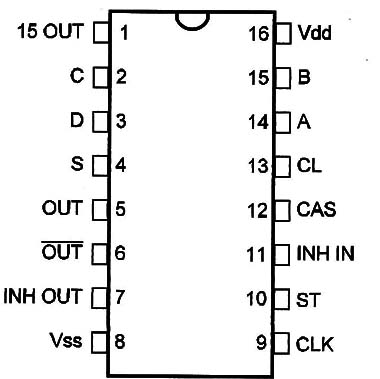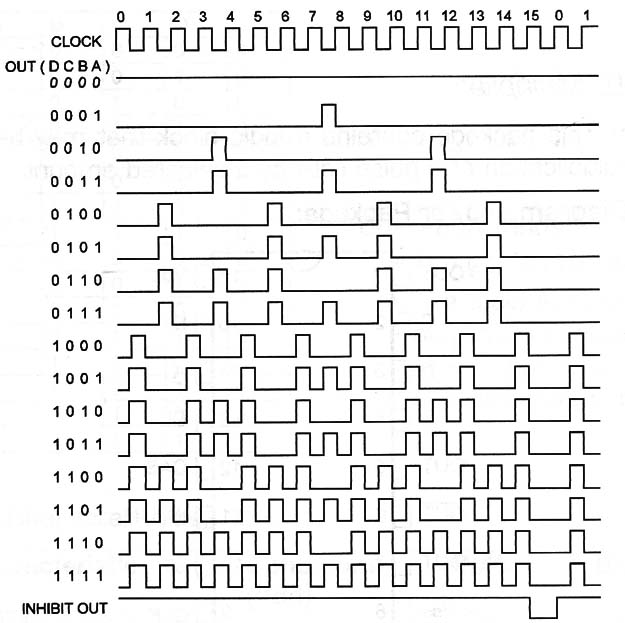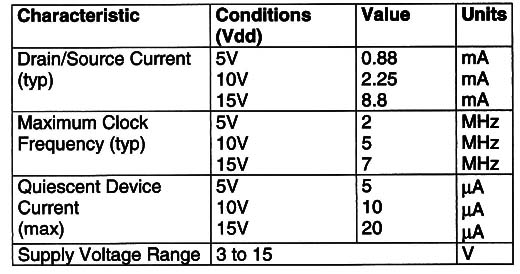### DatasheetsN° of component

## 4089 Binary Rate Multiplier (CM053E)

Description: This package contains a logic block that may be used to make the multiplication of a pulse rate by a selected amount.

Functional Diagram and/or Package:Pin Names

Vdd - Positive Supply Voltage (3V to 15V)

Vss - Ground

CL - Clear

S - Set to 15

15-OUT - 15 output

CLK - Clock

OUT, OUT/ - Output and Complementary Output

INH OUT - Inhibit Output

INH IN - Inhibit Input

ST - Strobe

A, B, C, D - Binary Input

Number (D = MSB)

Truth TableOperation Mode:

Pins 4, 1D, 11, 12, and 13 are grounded in normal operation.

Clock is applied to pin 9.

The binary number to Which the multiplication Will be made is applied to the inputs A, B, C, and D [D is the MSB].

Output is obtained at pin 6. The oomplement is found in pin 5.

The output is a signal with a frequency of one sixteenth of the Input frequency multiplied by the word presented to pins A, B, C, and D.

S and CL are used to synchronize the circuit.

INH IN stops the output pulses when put to 1.

Electrical Characteristics:Other Devices:

The 4527 is equivalent except for decimal rates.

Applications:

Instrumentation

Numerical Control

Digital Filters

Frequency Synthesis

Observations:

The output is average, Which means that the pulses are not equally spaced.

This device can be used in A/D and D/A converters.